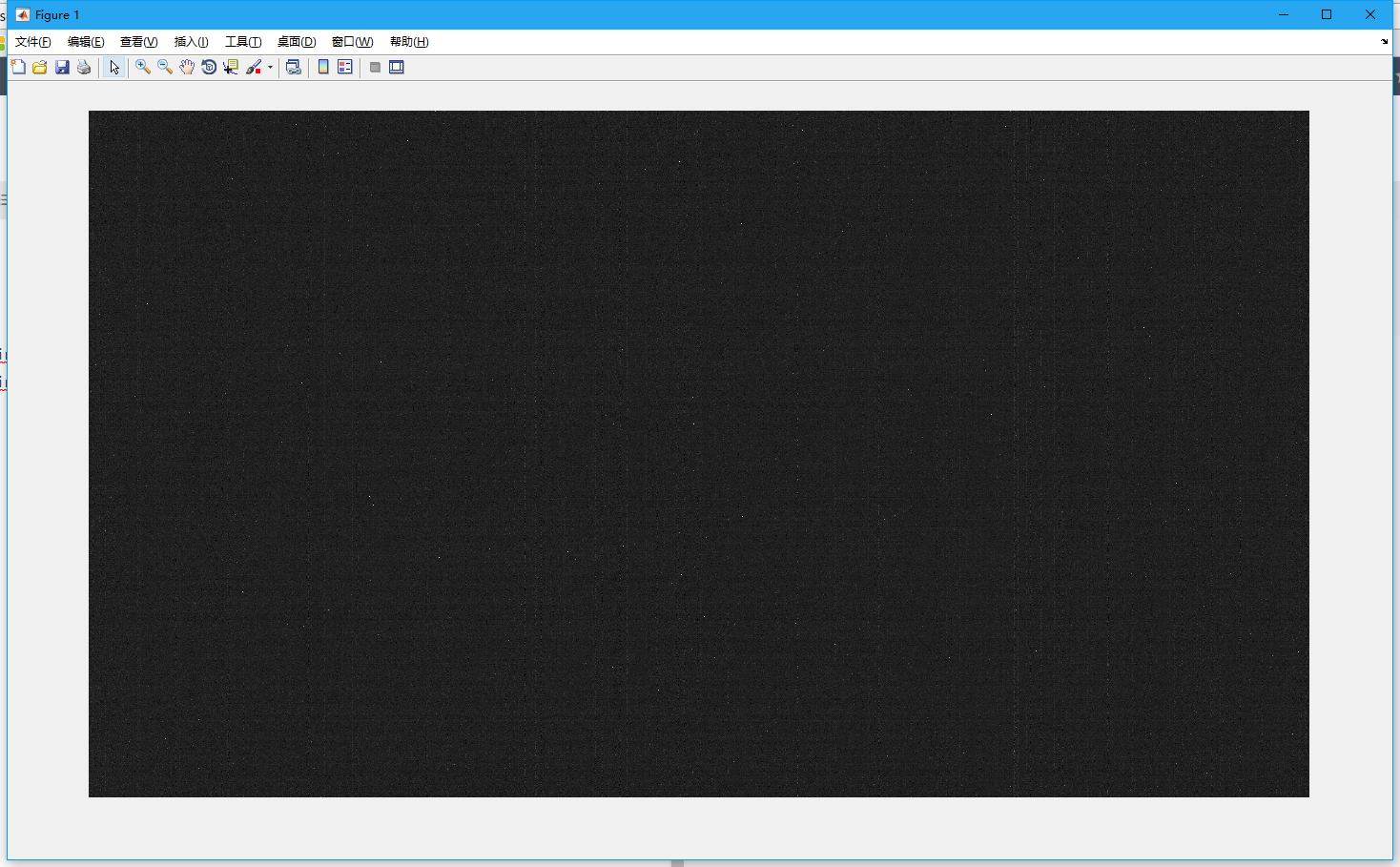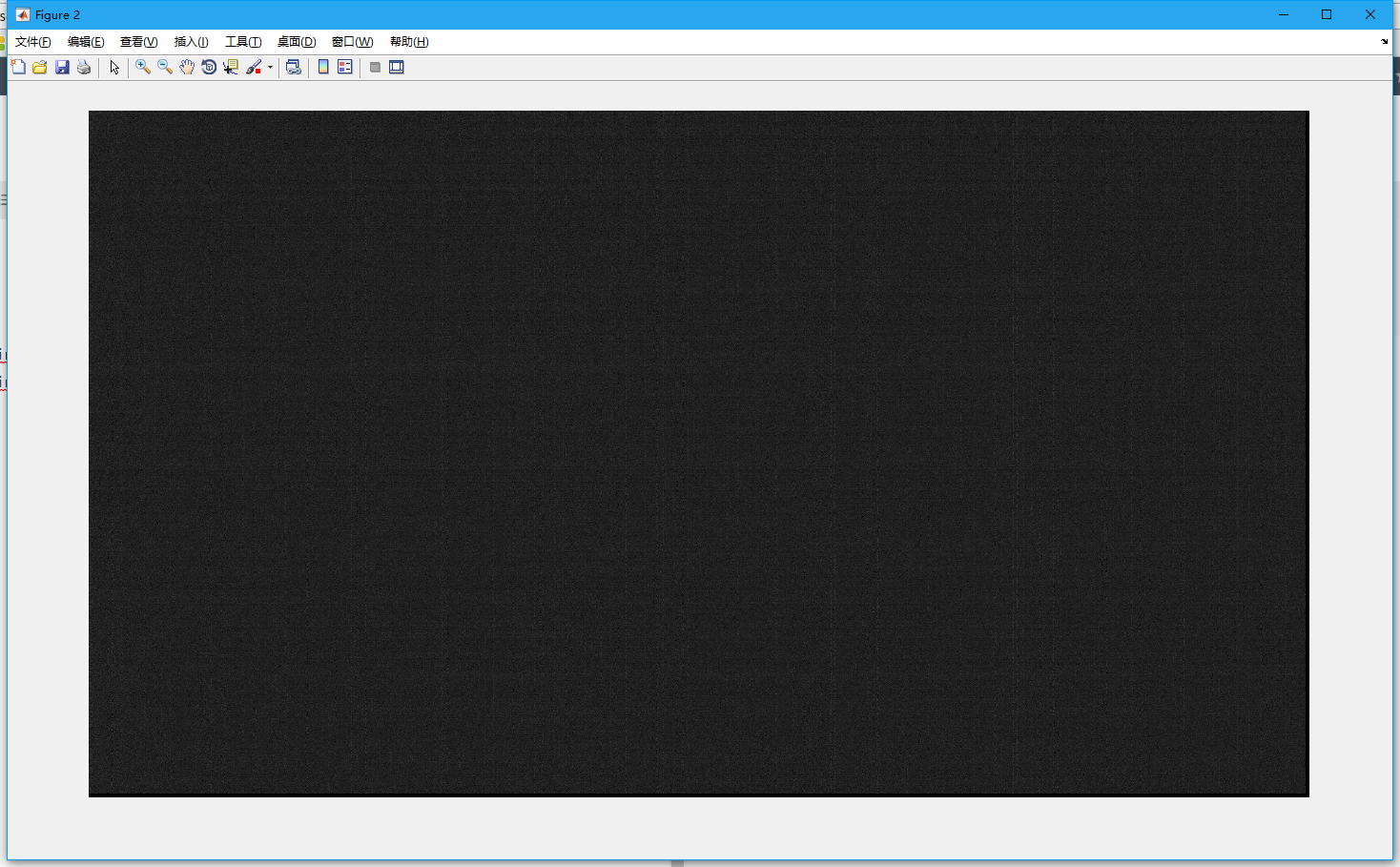# ISP – DPC

Time：2021-2-22

## introduce

In the image sensor, there are defects in the array technology formed by each light acquisition point on the sensor, or errors in the process of optical signal conversion, which will cause the information of some pixels on the image to be incorrect, resulting in inaccurate pixel values in the image. These defective pixels are bad pixels in the image.

Due to the different process technology and sensor manufacturers, especially for some low-cost, consumer sensor, there will be a lot of bad points. In addition, sensor in a long time, high temperature environment, there will be more and more bad points, thus damaging the image clarity and integrity.

Defect pixel correction is the way to solve this problem. The classification of defect points is as follows:

• Static bad points:

• Bright spot: Generally speaking, the brightness value of a pixel is proportional to the incident light, and the brightness value of a bright spot is significantly greater than the incident light multiplied by the corresponding proportion, and the brightness of the point will increase significantly with the increase of exposure time;
• Dark spot: no matter what incident light, the value of this point is close to 0;
• Dynamic bad point: in a certain range of pixels, the point is normal, and beyond this range, the point is brighter than the surrounding pixels. When the sensor temperature or gain increases, the dynamic bad point will become more obvious.

## reason

If there are bad points in the image, it will affect the surrounding pixels during interpolation and filtering, so it is necessary to correct the bad points before interpolation and filtering.

When there are many bad points or many dynamic bad points in the image, the false color will appear on the edge of the image. This phenomenon not only affects the clarity of the image, but also affects the color of the edge. In addition, bad points can also cause pixel flicker in the image.

## correcting

The correction method given here is based on Bayer image, and the principle of gray image is consistent.

Bayer format DPC method, the image is divided into R, G, B three images, respectively, for bad point correction. If the image format of sensor is rgbir, the image should be divided into four channels: R, GR, GB and B.

Dynamic and static correction are two independent processes.

## Static bad point correction

Static bad point correction is based on the existing static bad point table, comparing whether the coordinates of the current point are consistent with a coordinate in the static bad point table. If they are consistent, they are judged as bad points, and then calculate the correction results to correct them. The bad points of each sensor are different, so the sensor manufacturers need to give the static bad point table of each sensor. However, for the sake of cost, many sensor manufacturers do not give it, and users can only correct it one by one. Therefore, for some low-cost sensors, the practicability of static bad point correction is not very strong.

Due to the hardware design needs to occupy a lot of memory, considering the chip area and some other reasons, so the size of static bad points is limited, can not be unlimited correction.

Once the static bad point table of sensor is written into storage, the DPC module will automatically replace the bad points shown in the bad point table.

slightly

### tuning

The calibration method of each platform is different, but in general, the calibration is carried out according to the following steps:

• Mark the bright and the bad in the dark.
• Dark spots are calibrated in the environment of uniform light image.
• Merge the bad point table.

## Dynamic bad point correction

The correction of dynamic bad points can detect and correct the bright and dark points of sensor in real time, and the number of corrected bad points is not limited. Compared with static correction, dynamic correction has more uncertainty.

Dynamic DPC can be divided into two steps, namely, bad point detection and bad point correction.

### algorithm

Here I wrote a DPC algorithm, if you are interested, you can have a look.

This algorithm uses gradient percentage to detect the bad points. After the bad points are detected, the median filter is used to correct the bad points. Finally, the final calculation result is calculated by alpha mixing.

### code

``````close all;
clear;
clc;
%% variable
dp_slope = 0.02;
dp_thresh = -0.3;
r=3;        %Stencil radius

%% read raw image
% x = 0:255;
% y = dp_slope * x + dp_thresh;
% y(y<0) = 0;
% y(y>1) = 1;
% figure,
% plot(0:255,y)
% axis([0 255 0 1.5])

[filename, pathname] = ...
uigetfile({'*.raw'}, 'select picture');
str = [pathname filename];
fp = fopen(str, 'rb');
[X,l] = fread(fp, [1920,1080], 'uint16');
fclose(fp);
img = uint8(X/16)';
[height, width] = size(img);
img_correct = zeros(height, width);

%% Image edge extension
imgn=zeros(height+2*r,width+2*r);
imgn(r+1:height+r,r+1:width+r)=img;
imgn(1:r,r+1:width+r)=img(1:r,1:width);
imgn(1:height+r,width+r+1:width+2*r+1)=imgn(1:height+r,width:width+r);
imgn(height+r+1:height+2*r+1,r+1:width+2*r+1)=imgn(height:height+r,r+1:width+2*r+1);
imgn(1:height+2*r+1,1:r)=imgn(1:height+2*r+1,r+1:2*r);

%% dp algorithm
for i = r+1:height-r
for j = r+1:width-r

img_r = imgn(i-r:2:i+r, j-r:2:j+r);
data_r_center = img_r(r, r);
data_r_diff(1:r+1, 1:r+1) = abs(img_r - img_r(r,r));
data_r_sort = sort(img_r(:));
data_r_median = data_r_sort(r*2+1);
data_r_detect = data_r_diff * dp_slope + dp_thresh;
data_r_detect(data_r_detect < 0) = 0;
data_r_detect(data_r_detect > 1) = 1;
data_r_judge = sum(sum(data_r_detect > 0));
data_r_weight = sum(sum(data_r_detect)) / data_r_judge;
if i-r == 18 && j-r == 43
a = 1;
end
if data_r_judge >= 7
data_r_correct = data_r_median * data_r_weight + (1-data_r_weight) * data_r_center;
else
data_r_correct = data_r_center;
end
img_correct(i-r, j-r) = data_r_correct;

end
end

%% show
figure,imshow(uint8(img));
figure,imshow(uint8(img_correct));``````

### Effect display:### tunning

DPC debugging can improve many aspects of the image and improve the image quality to a great extent. In general, when different ISO are adjusted to different values, one is the mixing degree of blend, the other is the strength of bad point detection. In the case of more noise, the intensity of DPC can be enhanced, but it is recommended not to use DPC as denoising. If the DPC correction is too strong, it will still cause edge color deviation and overall color deviation.

## Emacs plug in ( two ) ——Persistent scratch

1、 Reference Contents of Emacs series articles — updating Re-open scratch buffer in Emacs? persistent-scratch Lisp Interaction Buffers 2scratch 2.1 basic introduction When startedeamcsWhen I was young, (1) It will enter automaticallyLISP interaction mode (2) Will automatically generate aLISP interactive buffer——scratch buffer 2.2 access*scratch* (1) C-x b *scratch* Execute the above command, it will enter […]